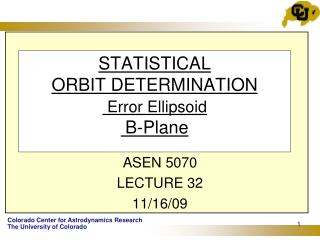DownloadDownload PresentationSTATISTICAL ORBIT DETERMINATION Error Ellipsoid B-Plane

# STATISTICAL ORBIT DETERMINATION Error Ellipsoid B-Plane

Download Presentation## STATISTICAL ORBIT DETERMINATION Error Ellipsoid B-Plane

- - - - - - - - - - - - - - - - - - - - - - - - - - - E N D - - - - - - - - - - - - - - - - - - - - - - - - - - -
##### Presentation Transcript

1. STATISTICALORBIT DETERMINATIONError EllipsoidB-Plane ASEN 5070 LECTURE 32 11/16/09

2. Example:

3. Views of Error Ellipsoid view (0,0)* azimuth =0, elevation =0 view down the negative y-axis *view(azimuth, elevation), azimuth is a clockwise rotation about the positive z-axis

4. Views of Error Ellipsoid view (90°,0) view down the positive x-axis

5. Views of Error Ellipsoid view (0,90°) view down the positive z-axis

6. Views of Error Ellipsoid view (-37.5°,0) standard matlab view

7. Body Plane (B-Plane)

8. Viking Launch Trajectory

9. Viking Trajectory

10. + OD solutions before L + 12h

11. Viking 1 Departure Control Uncertainty in time of closest approach 25=(212+122)1/2 3s Error ellipse for 1st mid course maneuver

12. Mars Climate Orbiter (MCO) B-Plane Mars Impact Radius CERTAIN DEATH AMD = Angular Momentum Desaturation

13. Error Ellipse in the B-plane I. Assume we have done an OD solution at some point, , in the interplanetary phase of the mission and we wish to project our estimation error covariance onto the B-plane (B=Body). • Compute the time of B-plane penetration based on conic motion (this is known as the linearized time of filter, LTOF) • Map P to this time using: • Generally P will be expressed in ECI, J2000 coordinates. Rotate the position portion of into the STR Frame

14. Error Ellipse in the B-plane 4. Use the 2x2 portion of corresponding to 5. Compute the Eigenvalues and normalized Eigenvectors 6. Compute the semi-major axis and semi-minor axis of the ellipse (x’,y’) and the rotation angle . Plot the 1,2, or 3 error ellipse on the B-plane. For a 2-D error ellipse the probability of being inside the N error ellipse (N=1,2,3…) is given by

15. Error Ellipse in the B-plane For a bivariate normal distribution: SMAA B-plane 7. The B-plane parameters are chosen because the variation of the and vectors are nearly linear with respect to midcourse orbit parameters.